# Finding an angle measure of a triangle given two angles Online Quiz

#### Complete Python Prime Pack

9 Courses     2 eBooks

#### Artificial Intelligence & Machine Learning Prime Pack

6 Courses     1 eBooks

#### Java Prime Pack

9 Courses     2 eBooks

Following quiz provides Multiple Choice Questions (MCQs) related to Finding an angle measure of a triangle given two angles. You will have to read all the given answers and click over the correct answer. If you are not sure about the answer then you can check the answer using Show Answer button. You can use Next Quiz button to check new set of questions in the quiz.Q 1 - Find the missing angle in the given triangle below. Sum of angles in a triangle is 180°.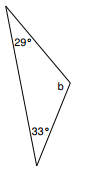### Explanation

Step 1:

Sum of angles in a triangle is 180°.

29° + 33° + b = 180°; 62° + b = 180°

Step 2:

b = 180° − 62° = 118°

So missing angle = 118°

Q 2 - Find the missing angle in the given triangle below. Sum of angles in a triangle is 180°.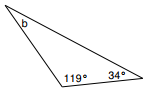### Explanation

Step 1:

Sum of angles in a triangle is 180°.

119° + 34° + b = 180°; 153° + b = 180°

Step 2:

b = 180° − 153° = 27°

So missing angle = 27°

Q 3 - Find the missing angle in the given triangle below. Sum of angles in a triangle is 180°.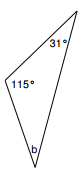### Explanation

Step 1:

Sum of angles in a triangle is 180°.

115° + 31° + b = 180°; 146° + b = 180°

Step 2:

b = 180° − 146° = 34°

So missing angle = 34°

Q 4 - Find the missing angle in the given triangle below. Sum of angles in a triangle is 180°.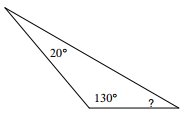### Explanation

Step 1:

Sum of angles in a triangle is 180°.

130° + 20° + x = 180°; 150° + x = 180°

Step 2:

x = 180° − 150° = 30°

So missing angle = 30°

Q 5 - Find the missing angle in the given triangle below. Sum of angles in a triangle is 180°.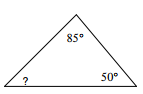### Explanation

Step 1:

Sum of angles in a triangle is 180°.

85° + 50° + x = 180°; 135° + x = 180°

Step 2:

x = 180° − 135° = 45°

So missing angle = 45°

Q 6 - Find the missing angle in the given triangle below. Sum of angles in a triangle is 180°.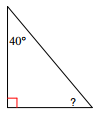### Explanation

Step 1:

Sum of angles in a triangle is 180°.

90° + 40° + x = 180°; 130° + x = 180°

Step 2:

x = 180° − 130° = 50°

So missing angle = 50°

Q 7 - Find the missing angle in the given triangle below. Sum of angles in a triangle is 180°.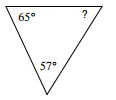### Explanation

Step 1:

Sum of angles in a triangle is 180°.

65° + 57° + x = 180°; 122° + x = 180°

Step 2:

x = 180° − 122° = 58°

So missing angle = 58°

Q 8 - Find the missing angle in the given triangle below. Sum of angles in a triangle is 180°.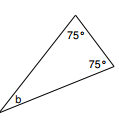### Explanation

Step 1:

Sum of angles in a triangle is 180°.

75° + 75° + b = 180°; 150° + b = 180°

Step 2:

b = 180° − 150° = 30°

So missing angle = 30°

Q 9 - Find the missing angle in the given triangle below. Sum of angles in a triangle is 180°.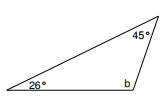### Explanation

Step 1:

Sum of angles in a triangle is 180°.

45° + 26° + b = 180°; 71° + b = 180°

Step 2:

b = 180° − 71° = 109°

So missing angle = 109°

Q 10 - Find the missing angle in the given triangle below. Sum of angles in a triangle is 180°.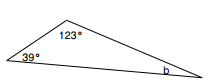### Explanation

Step 1:

Sum of angles in a triangle is 180°.

123° + 39° + b = 180°; 162° + b = 180°

Step 2:

b = 180° − 162° = 18°

So missing angle = 18°

finding_angle_measure_of_triangle_given_two_angles.htm